Next: Kepler Problem Up: Planetary Motion Previous: Kepler's Third Law

# Orbital Energies

According to Equations (215) and (223), the total energy per unit mass of an object in orbit around the Sun is given by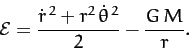(266)

It follows from Equations (247), (251), and (256) that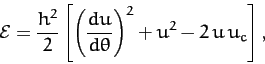(267)

where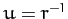, and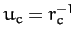. However, according to Equation (255),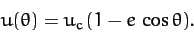(268)

The previous two equations can be combined with Equations (256) and (259) to give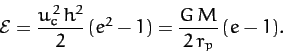(269)

We conclude that elliptical orbits (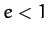) have negative total energies, whereas parabolic orbits (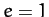) have zero total energies, and hyperbolic orbits (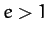) have positive total energies. This makes sense, since in a conservative system in which the potential energy at infinity is set to zero [see Equation (214)] we expect bounded orbits to have negative total energies, and unbounded orbits to have positive total energies--see Section 3.2. Thus, elliptical orbits, which are clearly bounded, should indeed have negative total energies, whereas hyperbolic orbits, which are clearly unbounded, should indeed have positive total energies. Parabolic orbits are marginally bounded (i.e., an object executing a parabolic orbit only just escapes from the Sun's gravitational field), and thus have zero total energy. For the special case of an elliptical orbit, whose major radius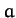is finite, we can write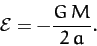(270)

It follows that the energy of such an orbit is completely determined by the orbital major radius.

Consider an artificial satellite in an elliptical orbit around the Sun (the same considerations also apply to satellites in orbit around the Earth). At perihelion,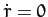, and Equations (266) and (269) can be combined to give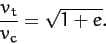(271)

Here,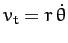is the satellite's tangential velocity, and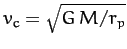is the tangential velocity that it would need in order to maintain a circular orbit at the perihelion distance. Likewise, at aphelion,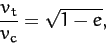(272)

where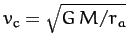is now the tangential velocity that the satellite would need in order to maintain a circular orbit at the aphelion distance.

Suppose that our satellite is initially in a circular orbit of radius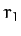, and that we wish to transfer it into a circular orbit of radius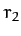, where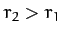. We can achieve this by temporarily placing the satellite in an elliptical orbit whose perihelion distance is, and whose aphelion distance is. It follows, from Equation (262), that the required eccentricity of the elliptical orbit is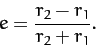(273)

According to Equation (271), we can transfer our satellite from its initial circular orbit into the temporary elliptical orbit by increasing its tangential velocity (by briefly switching on the satellite's rocket motor) by a factor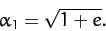(274)

We must next allow the satellite to execute half an orbit, so that it attains its aphelion distance, and then boost the tangential velocity by a factor [see Equation (272)]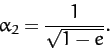(275)

The satellite will now be in a circular orbit at the aphelion distance,. This process is illustrated in Figure 19. Obviously, we can transfer our satellite from a larger to a smaller circular orbit by performing the above process in reverse. Note, finally, from Equation (271), that if we increase the tangential velocity of a satellite in a circular orbit about the Sun by a factor greater than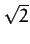then we will transfer it into a hyperbolic orbit (), and it will eventually escape from the Sun's gravitational field.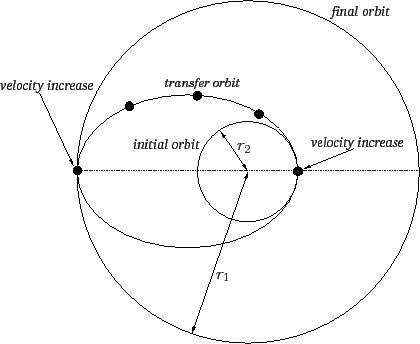Next: Kepler Problem Up: Planetary Motion Previous: Kepler's Third Law
Richard Fitzpatrick 2011-03-31